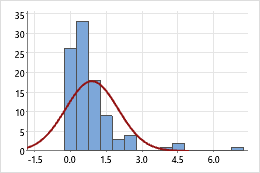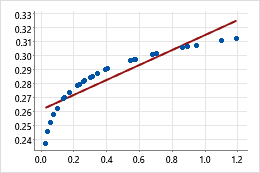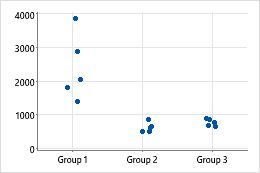# Log base 10 function

To use this function, choose Calc > Calculator.

Calculates the exponent to which 10 must be raised to equal a given number. For example, 102 = 100, so the log base 10 of 100 is 2. Log base 10 is defined only for positive numbers. When you multiply a number by 10, you increase its log by 1; when you divide a number by 10, you decrease its log by 1.

## Syntax

LOGTEN(number)

In number, specify the value or column of values. Minitab computes the value x such that 10x = the number. If you enter 0 or a negative number, Minitab stores a missing value *.

## Example

Calculator expression Result
LOGTEN(1000) 4

## Uses

In statistics, log base 10 (log10) can be used to transform data for the following reasons:
• To make positively skewed data more "normal"
• To account for curvature in a linear model
• To stabilize variation within groupsExample of transforming data to make it appear more normalIn the original graph, the data are positively skewed as shown by the values far out on the right (upper) tail. The log10 transformation compresses the upper tail and stretches out the lower tail, making the transformed data appear more normal.Example of transforming data to account for curvature in a linear modelIn the original scatterplot, the simple regression line does not accurately model the curvature in the data. After the X-scale is transformed using log10, the data values fall along the simple regression line.Example of transforming data to stabilize variation within groupsIn the original individual value plot, group 1 has larger values and thus appears to have greater within-group variability. After the data are transformed, the within-group variation appears similar.
By using this site you agree to the use of cookies for analytics and personalized content.  Read our policy